# Hunting in Synchronous Machines

## Hunting in Synchronous Machines

So far the operation of the synchronous machine under steady-state conditions at constant (synchronous) speed has been considered. Under these conditions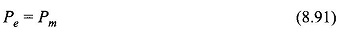where        Pe= electrical power output of the machine

Pm= mechanical power input to the machine

the machine losses having been assumed negligible. These power flows* are indicated in Fig. 8.65. If this power balance is disturbed, say for example, the mechanical power input slightly increases, the machine undergoes electromechanical dynamics wherein

P„,- Pe= Pa= accelerating power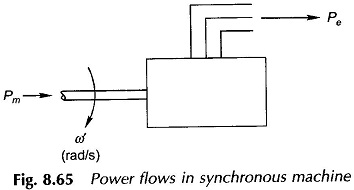In the absence of losses this power is absorbed in accelerating the rotor inertia, i.e.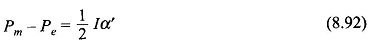where I = moment of inertia of rotor in kg m2

a’ = rotor acceleration in rad (meth)/s2

If powers are expressed in MW, Eq. (8.87) can be written as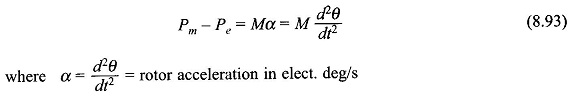M= inertia constant in MJ s/elect. deg; Pm,          Pe are in MW

It is convenient to measure the rotor angle with respect to a synchronously rotating reference frame. Let

where ws = synchronous speed in elect. deg/s

8= rotor angular displacement from the synchronously rotating reference frame; this in fact is the angle by which Ef leads V

Taking the second derivative of Eq. (8.94),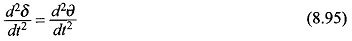Equation (8.92) therefore takes the form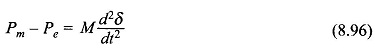It has already been shown that Pe, is governed by the power-angle characteristic of the machine, i.e.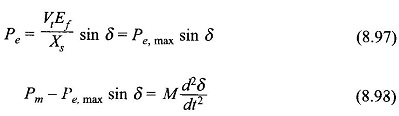It is a nonlinear second-order differential equation known as the swing equation. Let the machine be operating under steady conditions of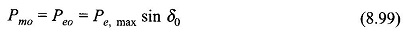With the mechanical input power remaining constant at Pmo. let the angle S be distributed from 80 to 30 + t18. ThenThe incremental change AS= 8– so is governed by the linear second-order differential equation (8.101) whose characteristic roots are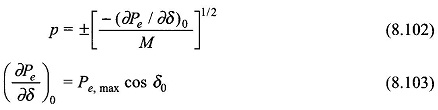From Eq. (8.101), (dPe/db)0 > 0 for S< 90°, i.e. in the stable* operating zone of the machine, the roots of the characteristic equation (8.101) are a complex conjugate pair indicating sinusoidal oscillatory behaviour about the operating point (Peo, 50).

Oscillatory behaviour of the synchronous machine about the operating point has its origin in machine stiffness (or the synchronous link between rotor and stator fields which has a spring-type action). This oscillatory behaviour known as hunting is highly undesirable—the electrical power fed to the mains and shaft torque oscillate, which in turn causes shaft fatigue. To minimize hunting to a tolerable level the oscillations must be damped out by introducing a damping term (proportional to d45 dt) in the swing equation (Eq. (8.98)). In fact a small amount of damping, contributed by system losses (both mechanical and electrical), is always present in the machine but this is insufficient to kill hunting. Additional damping must therefore be introduced in the machine.

## Damper Winding:

Additional damping is provided in the salient pole synchronous machine by means of damper bars located in the main poles of the machine and short-circuited through round rings at both ends as shown in Fig. 8.66. As the rotor oscillates, the damper bars have a relative movement with respect to the air-gap flux pattern which causes induction of emfs and flow of currents in these bars. The torque created by the bar currents as per Lenz’s law always opposes the relative motion. This is how a positive damping term is brought into play so that the oscillatory motion of the rotor about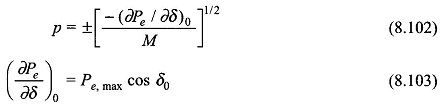the operating point is considerably reduced in amplitude and the rotor quickly returns to the steady position. These short-circuited bars are known as damper winding or ammortisseur winding. These act like the squirrel cage of an induction motor (refer Sec. 5.7) thereby providing a starting torque for the motor which otherwise being of synchronous kind is non-self-starting. Therefore, the damper winding serves the dual purpose.

## Synduction Motor:

A synduction motor started on the induction principle by means of damper winding is known as the synduction motor. On starting, the rotor achieves a speed close to synchronous and the rotor and stator fields lock into each other as soon as the field excitation is switched on.

Updated: April 18, 2018 — 11:20 am

`Please enable JavaScript in your browser to complete this form.Name *FirstLastEmail *Paragraph TextWebsite`
` `